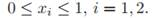# Write a decision-directed clusterer related to k-means in the following way. (a) First, generate a set D of n = 1000 three-dimensional points in the unit square, (b) Randomly choose c = 4 of these points as the initial cluster centers mj, j = 1, 2, 3, 4. (c) The core of the algorithm operates as follows: First, each sample xi, is classified by the nearest cluster center mj . Next, each mean mj is calculated to be the mean of the samples in ωj . If there is no change in the centers after n presentations, halt. (d) Use your algorithm to plot four trajectories of the position of the cluster centers. (e) What is the space and the time complexities of this algorithm? State any assumptions you invoke.

Question
1 views

Write a decision-directed clusterer related to k-means in the following way.

(a) First, generate a set D of n = 1000 three-dimensional points in the unit square,(b) Randomly choose c = 4 of these points as the initial cluster centers mj, j = 1, 2, 3, 4.

(c) The core of the algorithm operates as follows: First, each sample xi, is classified by the nearest cluster center mj . Next, each mean mj is calculated to be the mean of the samples in ωj . If there is no change in the centers after n presentations, halt.

(d) Use your algorithm to plot four trajectories of the position of the cluster centers.

(e) What is the space and the time complexities of this algorithm? State any assumptions you invoke.

### This question hasn't been answered yet.